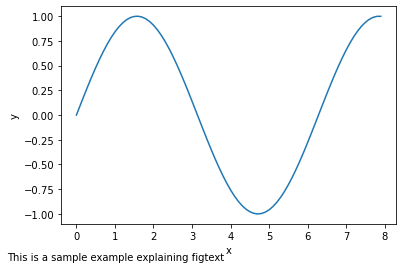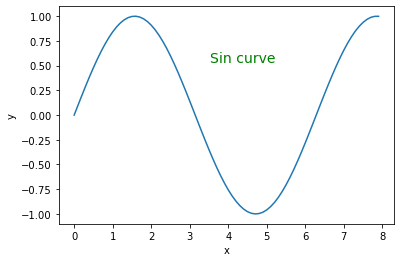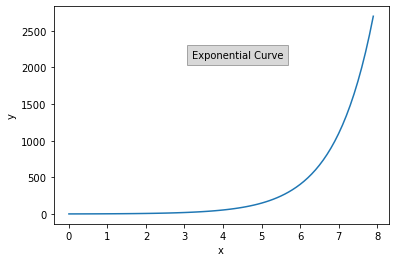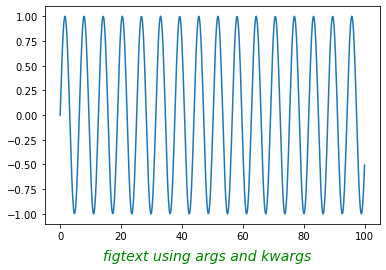Related Articles
Matplotlib.pyplot.figtext() in Python
• Last Updated : 19 Apr, 2020

Matplotlib is an extensively used Python library for data visualization. It is a multi-platform data visualization library built on NumPy arrays, also designed to work with the SciPy stack.

## Matplotlib.pyplot.figtext()

Figtext is used to add text to a figure at any location on it. You can even add the text outside the Axes. It uses the complete figure for co-ordinates, where bottom-left denotes (0, 0) and top right represents (1, 1). The centre of the figure is (0.5, 0.5).

Syntax:

`matplotlib.pyplot.figtext(x, y, s, *args, **kwargs)`
ParameterValuesUse
x, yFloatPosition to place the text. It is by default in figure coordinates [0, 1]
sStringText String

Example #1: A sample example demonstrating the use of figtext.

 `# importing required modules ``import` `matplotlib.pyplot as plt``import` `numpy as np``   ` `# values of x and y axes ``x ``=` `np.arange(``0``, ``8``, ``0.1``)``y ``=` `np.sin(x) ``plt.plot(x, y)`` ` `# pyplot.figtext(x, y, string)``plt.figtext(``0``, ``0``, "This ``is` `a sample example \``explaining figtext", fontsize ``=` `10``)`` ` `plt.xlabel(``'x'``) ``plt.ylabel(``'y'``) ``plt.show()`The above example places text on the bottom left of the figure of the given font size.

Example #2: We can also place text at a relative position in the figure by adjusting the values of x and y.

 `# importing required modules ``import` `matplotlib.pyplot as plt``import` `numpy as np``   ` `# values of x and y axes ``x ``=` `np.arange(``0``, ``8``, ``0.1``)``y ``=` `np.sin(x) ``plt.plot(x, y)`` ` `plt.figtext(``0.55``, ``0.7``,``            ``"Sin curve"``,``            ``horizontalalignment ``=``"center"``, ``            ``verticalalignment ``=``"center"``, ``            ``wrap ``=` `True``, fontsize ``=` `14``, ``            ``color ``=``"green"``)`` ` `plt.xlabel(``'x'``) ``plt.ylabel(``'y'``) ``plt.show()`Alignment arguments- horizontalalignment and verticalalignment place the text in the centre while the wrap argument makes sure that the text lies within the figure width. The color argument gives the font color.

Example #3: We can also add a bounding box around the text by using the bbox argument.

 `# importing required modules ``import` `matplotlib.pyplot as plt``import` `numpy as np``   ` `# values of x and y axes ``x ``=` `np.arange(``0``, ``8``, ``0.1``)``y ``=` `np.exp(x) ``plt.plot(x, y)`` ` `# pyplot.figtext(x, y, string)``plt.figtext(``0.55``, ``0.7``, ``            ``"Exponential Curve"``,``            ``horizontalalignment ``=``"center"``,``            ``wrap ``=` `True``, fontsize ``=` `10``, ``            ``bbox ``=``{``'facecolor'``:``'grey'``, ``                   ``'alpha'``:``0.3``, ``'pad'``:``5``})`` ` `plt.xlabel(``'x'``) ``plt.ylabel(``'y'``) ``plt.show()`Example #4: We can also use *args and **kwargs to add text properties to our plot. *args and **kwargs are used to pass multiple arguments or keyword arguments to a function.
Note: For more info refer to the article: *args and **kwargs in Python

 `# importing required properties``import` `numpy as np``import` `matplotlib.pyplot as plt`` ` `x ``=` `np.linspace(``0``, ``100``, ``501``)``y ``=` `np.sin(x)`` ` `figtext_args ``=` `(``0.5``, ``0``,``                ``"figtext using args and kwargs"``)`` ` `figtext_kwargs ``=` `dict``(horizontalalignment ``=``"center"``, ``                      ``fontsize ``=` `14``, color ``=``"green"``, ``                      ``style ``=``"italic"``, wrap ``=` `True``)`` ` `plt.plot(x, y) ``plt.figtext(``*``figtext_args, ``*``*``figtext_kwargs)``plt.show()`Attention geek! Strengthen your foundations with the Python Programming Foundation Course and learn the basics.

To begin with, your interview preparations Enhance your Data Structures concepts with the Python DS Course. And to begin with your Machine Learning Journey, join the Machine Learning – Basic Level Course

My Personal Notes arrow_drop_up